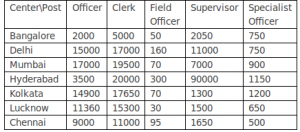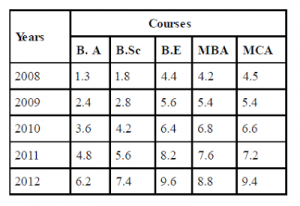## Data Interpretation For SBI PO : Set – 37

D.1-5) Study the following table to answer the given questions:1) In Kolkata number of Specialist officer is  approximately what percent of that officer?

a) 8.7

b) 9

c) 6.5

d) 8

e) 6.9

d)

In Kolkata :

No. of Officers = 14900

No. of Specialist Officers = 1200

Required  percentage = 1200 x 100/15000 = 8

2) Which center has 300% more number of clerks as compared to Bangalore?

a) Luck now

b) Mumbai

d) Chennai

e) None of these

c)

5000 + 300% of 5000 = 20000

3) What is the difference between total number for officers and Clerks?

a) 29, 680

b) 34, 180

c) 32, 690

d) 28, 680

e) None of these

c)

Total no. of officers = 2000 + 15000 + 17000 + 3500 + 14900 + 11360 + 9000 = 72,760

Total no. of clerks = 5000 + 17000 + 19500 + 20000 + 17650 + 15300 + 11000 = 105, 450

∴ Required difference = 105, 450 – 72, 760 = 32, 690

4) In Chennai number of clerks is approximately how much percent more than that of officers?

a) 18

b) 22

c) 20

d) 2

e) 13

b)

In chennai

No. of clerks = 11000

No. of officers = 9000

∴ Required percentage excess

= 11000 – 9000/9000 x 100

= 22.2 = 22

5) Which center has the highest number of candidates?

a) Delhi

b) Kolkata

d) Mumbai

e) None of these

d)

Number of candidates in

Delhi = 15000 + 17000 + 160 + 11000 + 750 = 43, 910

Mumbai = 17000 + 19500 + 70 + 7000 + 900 = 44, 470

Hyderabad = 3500 + 20000 + 300 + 9000 + 1150 = 33, 950

Kolkota = 14900 + 17650 + 70 + 1300 + 1200 = 35, 120

Mumbai has the highest number of candidates.

D.6-10) Study the table carefully to answer the questions that follow :

Yearly fees (in Rs. thousands) of five different courses in five different years :6) What was the difference between the total yearly fees for all the courses together in the year 2012 and the yearly fees of B.Sc course in the year 2010 ? (in Rs)

a) 34240

b) 28300

c) 37200

d) 25350

e) None of these

c)

6.2+7.4+9.6+8.8+9.4) – (4.2) = 37200

7) If 30% of the yearly fees were reduced for B.E course in the year 2011, what was the rectified yearly fees for B.E course in the year 2011 ?

a) Rs. 2460

b) Rs. 5740

c) Rs. 6260

d) Rs. 3230

e) None of these

b)

Required Fees = 8200 * 70/100 = Rs.5740

8) Total yearly fees for MBA course overall the years together was what percentage of total yearly fees of MCA course in the year 2010, 2011 and 2012 together ?

a) 70.73%

b) 104.46%

c) 87.26%

d) 141.38%

e) None of these

d)

(4.2 + 5.4 + 6.8 +7.6 + 8.8)/ (6.6 + 7.2 + 9.4) * 100
= 32.8/23.2 * 100 = 141.38%

9) What was the average yearly fees of MCA course overall the years together ?

a) Rs. 7120

b) Rs. 6620

c) Rs. 5940

d) Rs. 6460

e) None of these

b)

=  (4.5 + 5.4 + 6.6 + 7.2 + 9.4)/5
= Rs. 6620

10) What was the percentage increase in yearly fees of B. A in the year 2009 as compared to the previous year ?

a) 84 8/13%

b) 45 7/24%

c) 61 5/24%

d) 26 2/13%

e) None of these